# 前言

1.掌握栈的特点及其描述方法
2.掌握链式存储结构实现一个栈
3.掌握链栈的各种基本操作
4.掌握栈的典型应用的算法

1.设计一个选择式菜单。
2.设计一个整型数据元素的链栈。
3.编写入栈、出栈和显示栈中全部元素的程序。
4.编写一个把十进制数转换成八进制数的应用程序。

# 一、栈的顺序结构及其实现

## 1：顺序栈概念理解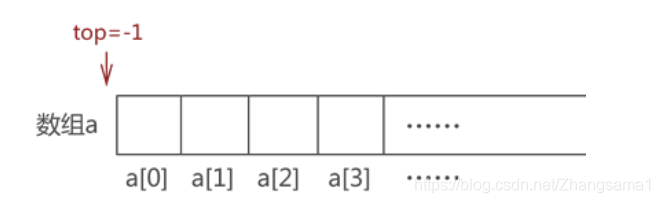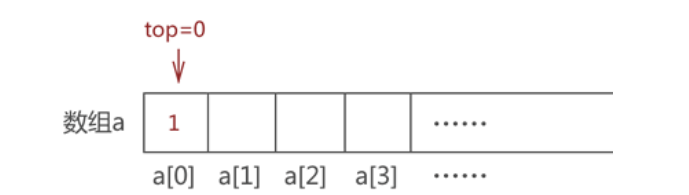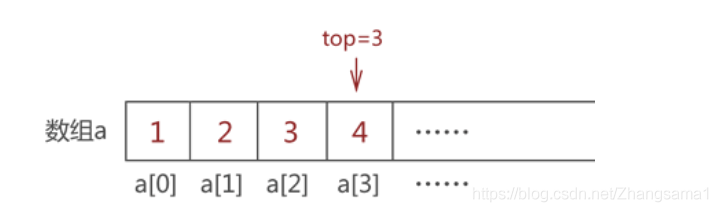## 2：入栈(压栈)实现方法

``````#pragma once
#include<iostream>
#include <cstdio>
#include<cstdlib>
typedef int Status;
typedef int ElemType;
#define TRUE 1
#define FALSE 0
#define OK 1
#define ERROR 0
#define MAXSIZE 10
#define make (struct student*)malloc(sizeof(struct student));
using namespace std;
typedef struct
{

ElemType stack[MAXSIZE];//构建一个顺序栈存储元素
int top;		//默认值为-1而不是0 -1代表代表的是栈空
}Stack;

Status push_stack(Stack* s, ElemType e) //入栈
{

if (s->top >= MAXSIZE - 1) //栈满退出
return ERROR;
s->top++;   //增加一位
cout << "要插入的数据是" << e << endl;
s->stack[s->top] = e;//赋值
return OK; //返回成功结果
}
``````

## 3：出栈(弹栈)及其实现方法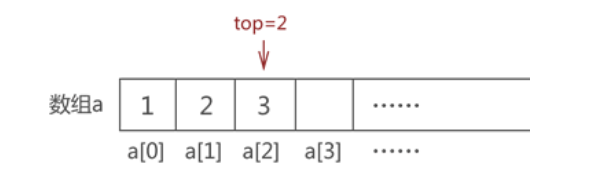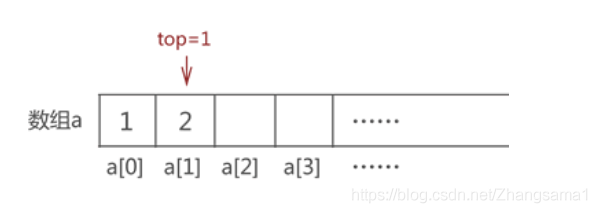``````
Status pop_stack(Stack* s, ElemType* e) //出栈
{

if (s->top == -1) //空栈
return ERROR;
*e = s->stack[s->top]; //取得要删除的值 这里没有-1是因为栈的起始数量是-1哦
cout << "要删除的数据是" << *e << endl;
s->top--;  //弹栈
return OK;
}
``````

## 4：顺序栈输出其数据

``````void show_stack(Stack s)
{

int j;
j = s.top;  //得到目前已有的数据个数
while (j > -1)
{

cout << "第"<<j<<"个元素是" << s.stack[j] << endl;
j--;
}
cout << "栈已空" << endl;
}
``````

## 5：主函数

``````int main()
{

head->top = -1;//初始top指针为-1 代表空栈
ElemType e;
}
``````

# 二、栈的链式存储结构

## 2.初始化链栈

``````#pragma once
#include<iostream>
#include <cstdio>
#include<cstdlib>
typedef int Status;
typedef int ElemType;
#define TRUE 1
#define FALSE 0
#define OK 1
#define ERROR 0
#define MAXSIZE 10
#define make (struct student*)malloc(sizeof(struct student));
using namespace std;

typedef struct StackNode
{

ElemType data;  //数据
int count; //记录当前栈内数据个数
struct StackNode* next; //指向下一个节点指针
}StackNode;

StackNode* init_stack(ElemType e)
{

StackNode* head = (StackNode*)malloc(sizeof(StackNode));//s是指向结构体StackNode的指针
cout << "栈底元素为" << e << endl;
head->count = 1;	//初始化数据数量为1,头指针自身也算一个数据
}
``````

## 3.链栈元素入栈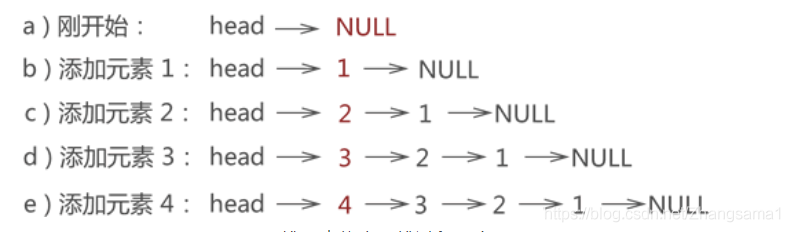``````
StackNode* push_stack(StackNode* head, ElemType e)//入栈 在栈L中插入数据e
{

StackNode* s = (StackNode*)malloc(sizeof(StackNode));//s是指向结构体StackNode的指针
s->data = e; //添加数据e
cout << "插入元素" << e << endl;
cout <<"当前元素个数为" << s->count << endl;
s->next = head; //指向上一元素
head = s; //头指针指向栈顶
}
``````

## 4.链表元素出栈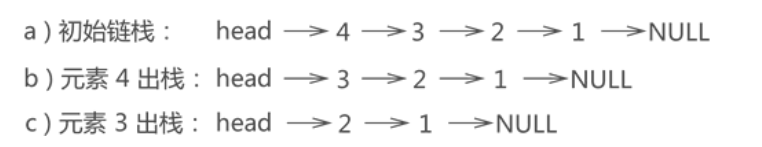``````StackNode* pop_stack(StackNode* head, ElemType* e)
{

StackNode* p;	//临时指针
if (head == NULL) //判断链栈非空
return ERROR;
*e = head->data; //取得要删除元素 也就是栈顶元素
cout << "要删除的元素是" << *e << endl;//输出要删除的元素
p = head->next; //p指向将被删除数据的下一个节点，以便头指针移位
head = p;	//头指针指向新位置
cout << "当前元素个数为" << head->count << endl;
}
``````

## 5.显示链栈元素

``````void show_stack(const StackNode *head)
{
int num = head->count;//取得元素个数
for (int i = 1; i <= num; i++)
{

cout << "第" << i << "个元素是" << head->data<<endl;//输出数据 从上至下输出
}
}
``````

## 6.主函数

``````
int main()
{

ElemType e;

}
``````

# 三、栈子系统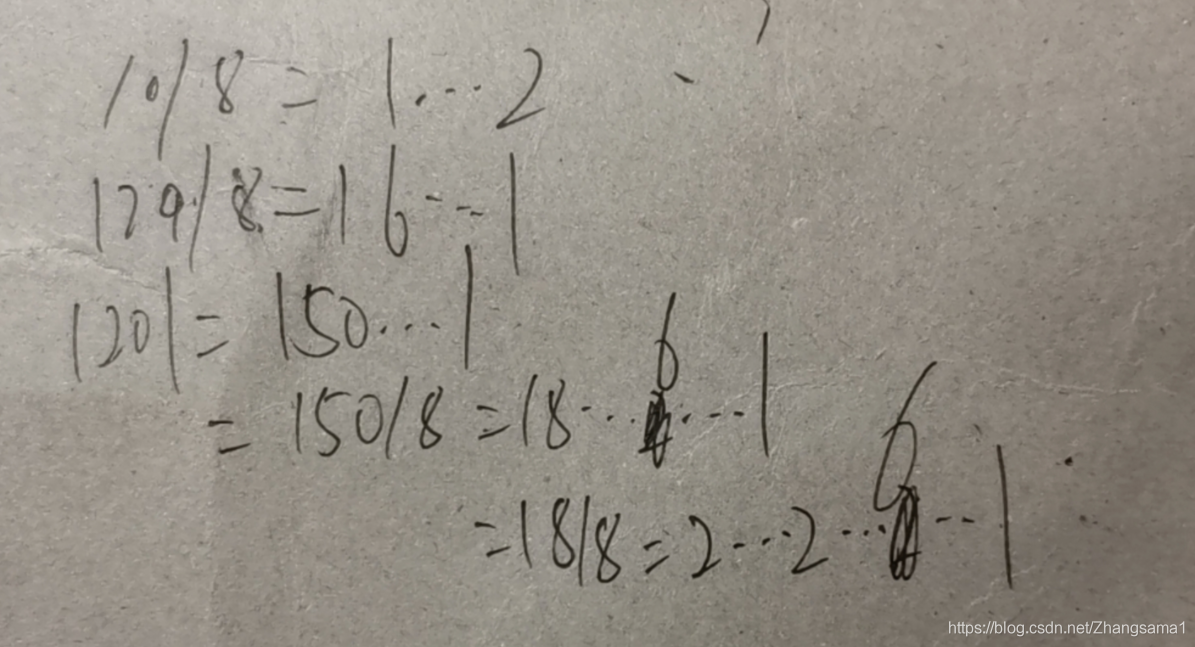``````void change(int m) //用递归来将十进制换为八进制
{

if (0 == m)
return;
int tmp = m % 8;
m = m / 8;
change(m);
printf("%d", tmp);

}
``````

``````#include <algo.h>
typedef struct StackNode
{

ElemType data;  //数据
int count; //记录当前栈内数据个数
struct StackNode* next; //指向下一个节点指针
}StackNode;

StackNode* init_stack(ElemType e)
{

StackNode* head = (StackNode*)malloc(sizeof(StackNode));//s是指向结构体StackNode的指针
cout << "栈底元素为" << e << endl;
head->count = 1;	//初始化数据数量为1,头指针自身也算一个数据
}
StackNode* push_stack(StackNode* head, ElemType e)//入栈 在栈L中插入数据e
{

StackNode* s = (StackNode*)malloc(sizeof(StackNode));//s是指向结构体StackNode的指针
s->data = e; //添加数据e
cout << "插入元素" << e << endl;
cout <<"当前元素个数为" << s->count << endl;
s->next = head; //指向上一元素
head = s; //头指针指向栈顶
}
StackNode* pop_stack(StackNode* head, ElemType* e)
{

StackNode* p;	//临时指针
if (head == NULL) //判断链栈非空
return ERROR;
*e = head->data; //取得要删除元素 也就是栈顶元素
cout << "要删除的元素是" << *e << endl;//输出要删除的元素
p = head->next; //p指向将被删除数据的下一个节点，以便头指针移位
head = p;	//头指针指向新位置
cout << "当前元素个数为" << head->count << endl;
}
void show_stack(const StackNode *head)
{
int num = head->count;//取得元素个数
cout << "元素总个数为" << num << endl;
for (int i = 1; i <= num; i++)
{

cout << "第" << i << "个元素是" << head->data<<endl;//输出数据 从上至下输出
}

}
void change(int m) //用递归来将十进制换为八进制
{

if (0 == m)
return;

int tmp = m % 8;
m = m / 8;
change(m);
printf("%d", tmp);

}
{

int times = 1;
cout << "元素个数" << head->count << endl;
int num = head->count;//取得当前元素个数
for(times;times<=num;times++)
{

cout << endl;//输出换行符
}
}
int main()
{

int choose;
ElemType e;
cout << "输入栈底元素" << endl;
cin >> e;
StackNode* head = init_stack(e);//初始化链栈
cout << "*******************" << endl;
cout << "*1 ……入栈       *" << endl;
cout << "*2 ……出栈       *" << endl;
cout << "*3 ……显示       *" << endl;
cout << "*4 ……数制转换   *" << endl;
cout << "*0 ……返回       *" << endl;
cout << "*******************" << endl;
while (1)
{

cout << "请选择功能" << endl;
scanf("%d", &choose);
switch (choose)
{

case 1:
{

ElemType e;
cout << "输出要插入的数据" << endl;
cin >> e;
cout << "插入成功" << endl;
break;
}
case 2:
{

ElemType e;
cout << "删除成功" << endl;
break;
}
case 3:
{

break;
}
case 4:
{

break;
}
case 0:
{

cout << "程序结束" << endl;
system("pause");
exit(0);
//return 0;
}
default:
break;
}
}
}
``````

# 四、链栈与顺序栈优缺点对比

### 福建师范大学计算机科学与技术研究生复试,2020福建师范大学考研复试内容及复试方式 考研复试流程..._weixin_39731107的博客-程序员宝宝

2020福建师范大学考研复试内容及复试方式是什么样的？根据福建师范大学2019年硕士研究生招生复试录取办法中提到，福建师范大学研究生复试学院根据学科(类别)、专业(领域)特点及办学特色确定复试内容和复试方式。福建中公教育小编给考生整理如下：(一)主要内容1.专业素质和能力(1)大学本科阶段学习情况及成绩。(2)全面考核考生对本学科(类别)、专业(领域)理论知识和应用技能的掌握程度，利用所学理论发现...

### Java Web 发送和接收数据servlet中文乱码问题 pageEncoding与charset的作用，response和request的setCharacterEncoding 的作用。_蓝盒子itbluebox的博客-程序员宝宝

Java Web 发送数据中文乱码问题 pageEncoding与charset的作用，response和request的setCharacterEncoding 的作用。在jsp代码中的头部往往有这两行代码&lt;%@ page language="java" contentType="text/html; charset=UTF-8" pageEncoding="UTF-8"%&g...

### AJAX框架汇总_yanick的博客-程序员宝宝

引此文原出于AJAX Patterns网站的一篇《Ajax Frameworks》的wiki文章，很早前我就注意到，后来在国内也有人翻译了，不过最近发现此wiki还是在不断添加维护中，截止此文发布前，作者又添加了好几个新诞生的AJAX开发工具，所以我决定重新翻译一遍，并且时常注意原文发布状态，一有新的内容立马也翻译过来，做到同步:)此翻译稿很大一部分内容出自国内出现的那个先前版本，我

### java fx 给按钮加颜色_JavaFx：9、Button按钮以及简单介绍设置背景颜色和外边框等问题..._sxtybzwm的博客-程序员宝宝

package fx.com;import javafx.application.Application;import javafx.event.ActionEvent;import javafx.event.EventHandler;import javafx.geometry.Insets;import javafx.scene.Group;import javafx.scene.Scene;...